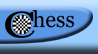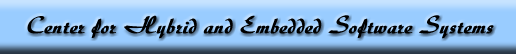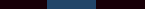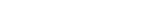Workspaces ---- 337staff act actionwebs actionwebslocal agv apesless apon automotive bcvtb bhc bipeds capecode certafcs cg chess chessadmin chesslocal chessworkshop clotho cosmoi cps cpslab croquet cyphysim dataflow ddosos denso densoeal densosas design dgc3 dgc3exec directors drosonetsim dsc eecs124 eecs149 eecs149lab eecs20 escher gm gsoc handsimdroid hcddes hcssas hilv hybrid hyper iab ieee1588 instructors mast metropolis modelica naomi nes nsferc pa presenters pret pss ptalon ptango ptconf ptdb ptemaf ptesdf ptexternal pthomas ptides ptidesarch ptolemy ptopenmodelica ptpresenters pturing reactable reap researchers scos softdevel softwalls spfsos superb tbd thales triq vw# Student Progress—

[ home ][ students ][ projects ][ progress ][ mentors ][ overview ][ chess ]

#### Visit the projects page for downloads of the final posters and papers.rdgregg@eecs.berkeley.edu

# Robert Gregg

## Hybrid Reduction of a Passive Bipedal Walker from Three to Two DimensionsWeek 1

During the first week, I began an intensive literature search on topics related to bipedal walkers, classical mechanics, robotic manipulation, and Lagrangian formulations. Some useful papers were Simplest Walking Model: Stability, Complexity, and Scaling (Garcia), Compass-like biped robot Part I: Stability and bifurcation of passive gaits (Goswami), etc, and some useful books were Fundamentals of Mechanics of Robotic Manipulation (Ceccarelli) and A Mathematical Introduction to Robotic Manipulation (Murray, Li, Sastry).

I also performed some exercises on Lagrangian formulations to test my understanding of this concept. I was successful in deriving the Lagrangian and equations of motion for a single pendulum (2D and 3D), a double pendulum (2D), and an inverted pendulum on cart (2D), which can all be found here. I began to derive the model for a simple bipedal walker but was unfinished as of Friday.

Week 2

At the beginning of the week, I decided to adopt Goswamis generalized model, which parameterizes the position of the leg masses, as opposed to Garcias fixed foot mass. I then finished re-duplicating the generalized bipedal walker model given in Goswami. I noticed one inconsistency: my potential energy formula is dependent on the slope angle, similar to Garcias model, whereas Goswamis potential formula does not consider the slope angle.

After formulating the dynamics of the walker, I began studying the hybrid transition at the walkers impulse foot impact. Using Goswami and Stability of Hybrid System Limit Cycles: Application to the Compass Gait Biped Robot (Hiskens), I developed a strategy to finding stable limit cycles using the methods of Poincaré.

Aaron, Haiyang, Simon, and I met to discuss the simulation phase of this project. We briefed Haiyang and Simon on the project and decided to first re-duplicate the results of Goswami before attempting the 3D model, which does not have a known model analysis to check. I confirmed my strategy with Aaron and gave my 2D walker model to Simon for implementation in Hy-Visual.

Week 3

I made great progress on the modeling aspect of my project. I began my 3D biped model on paper, but quickly discovered that it is far too complicated to handle by hand. I spent a day learning how to use Mathematica, specifically for the purpose of symbolic simplification. Using this tool, I finished my 3D biped model, which includes the Lagrangian and normalized, generalized equations of motion. I tested the models correctness by fixing the x-axis angles to 90 degrees, which fixes the system in two dimensions, and verified that the Lagrangian simplifies to the previously derived formula.

Furthermore, with the help of Haiyang, I learned that the potential energy is independent of the slope angle because my angle-state system implicitly includes the slope. Garcia uses a different angle system that does not. Furthermore, during transition the non-stance angle minus the stance angle equals the opposite of the slope, so this further displays the relationship.

Having the 3D model, Aaron and I discovered that the Lagrangian is not immediately independent of any of the x-axis angles. This contradicts our original hypothesis and makes the reduction non-trivial. In the next week, I will attempt to get around this problem with a few tricks: making the hip mass much larger than the leg masses such that M/m approaches infinity, linearizing the Lagrangian, and trying a different walker structure. Aaron is going to continue working on the impact equations, which are also needed for the hybrid reduction.

A future path we might take in this project is to use Grizzle's generalized coordinate system from Asymptotically Stable Walking for Biped Robots: Analysis via Systems with Impulse Effects. This coordinate system includes the angle states from the current coordinates as well as the cartesian coordinates of the pivot foot. Aaron believes this will assist his impact equation derivation, so I may re-derive the 3D model in this coordinate system at a later date.

Week 4

I was successful in completely eliminating some of the Lagrangian dependencies using the following two techniques:
• fixing the angle 2*alpha between the walker's legs; I found one cyclic variable, phis[t]
• taking the limit as M/m approaches infinity; I found three cyclic variables. The Lagrangian is now in terms of three variables, phis[t], phis'[t], and thetas'[t] (a significant simplification of the formula!)

With these simplifications, the dynamics of the model are ready for the reduction. Aaron and I are still unfinished with the 3D impact equations, so this week I began researching some papers on them, including the previously mentioned Grizzle paper and Rigid Body Collisions of Planar Kinematic Chains with Multiple Contact Points (Hurmuzlu, Marghitu). It appears that generalized coordinates will be very useful for the impact equation aspect of the model.

Also, Haiyang, Simon, and I met and discussed the simulation and animation part of the project. They are practically done with simulation; they just need to find some initial values that produce asymptotically stable walking cycles. Simon is still working on the animation, which will be a visualization of the simulations. Beyond that, both Haiyang and I proved the following 2D-model relation that holds at all times:
thetas[t]  thetans[t] = alpha[t]
where alpha is the angle between the legs. This confirms my original assumption that the dynamics formula is not dependent on alpha so long as the two leg angles are included. Haiyang further found a relation that describes the double-support phase impact condition:
thetans[t] + thetas[t] = 4pi  2phi and thetans[t] > thetas[t]
where phi is the slope angle. Following this, we made an interesting observation: the relation given in Goswami to describe the impact condition of the Poincare's fixed point, phi = -0.5(thetans[t] + thetas[t]), appears to be wrong. We cannot get the geometry to work, particularly with the example where slope is set to zero (the leg angles should be complimentary but that is contradicted in this relation).

My goals for next week include finishing the impact equations with Aaron so that I can begin reducing the hybrid model. Moreover, Haiyang encouraged me to search for a closed-form approximation to determine the initial conditions that lead to asymptotically stable walking cycles. I have not read of any such method, except by linearizing the system (a discouraged approach), so such a discovery would be novel. At first glance, I cannot think of a feasible strategy because of the non-linearity of the system (cannot use linear algebra, Laplace transforms, etc). I am going to put some thought into the problem.

• Week 5

I put some thought into the closed-form initial state problem, and I am once again convinced that it is impossible due to the characteristics of the system. The only possibility is linearizing the system and solving backwards for an approximation, but this only holds for points close to the origin. Therefore, I am sure that a phase space search is the best approach.

Upon further examination, I realized that my mass ratio simplification, while correct for the stance leg equations of motion, undesirably eliminated the equations of motion for the non-stance leg. This is because I divided through by µ on the rows of the system that are not dependent on the mass ratio, which are the rows that describe the non-stance leg. Therefore, by taking the limit as the ratio approaches infinity, I destroyed the equations of motion. In order to address this issue, I applied the simplification to the stance leg rows (two and four) and left the other rows alone. This, however, led to a system without cyclic variables.

Aaron and I attempted another simplification method by fixing the theta angles to be equal. Conceptually, this means the top-down view of the robot resembles a straight line. This gave us very optimistic results: both theta angles are cyclic and the theta derivative terms combine nicely (the derivatives are also equal). Furthermore, we found that the phi rows in the kinematics matrix M are exactly the same as the rows in the 2D models matrix. More precisely, the 2D models matrix M is the submatrix M1 in the top-left corner of the simplified 3D models matrix M; the bottom-right submatrix M2 describes the variables introduced in the 3D model. This shows that the reduced 3D model is simply an augmented version of the 2D model!

Aaron provided the Routhian reduction of my simplified model, and I derived the reduced equations of motion using the same procedure as before. I will be working closely with Simon to get the original 2D model simulation working, including the search for stable limit cycles. Once that is finally complete, we will implement the reduced 3D model and hopefully have some positive results.

Finally, I am preparing a presentation of my research for next weeks CHESS meeting. Although I am not done with the project, I will report my progress and future goals. I also finished a preliminary abstract for the paper.

Week 6

Upon finishing the reduced model, I came to a couple conclusions. Matrices M and F and vector g remain the same as the original 2D model, the only difference is an additional augmented term. Also, the reduced model is a more general version of the original model, since setting Routhian constant c to zero results in the original 2D model. As constant c increases, the velocity in the x-y plane of the walking increases. Finally, we can uniquely bring the model back into three dimensions using the Routhian relation with theta prime. I suggest looking at my CHESS presentation for details and examples.

As of now, we have been unable to reproduce the original 2D models limit cycles, as found by Goswami, but this is somewhat expected because of the extreme sensitivity of the model to parameter and variable changes. We need an algorithm to search the state space over infinitesimal changes in order to find the limit cycles, and I have already started thinking ways to do this. Once HaiYang decides the best way to do this with Ptolemy II, I will begin coding it.

I implemented the reduced model in Ptolemy II as well. Similarly, I have not yet found limit cycles, but I did confirm my observations about the model. As I increase constant c, the robot seems to have more weight and falls over without increased initial angular velocity. This intuitively makes sense, one, because the augmented term is part of the potential component of the system and thus imitates a gravitational force, and two, because the inertia of the high velocity movement in the x-y plane will hold back the robot.

We are nearing the end of the project. Once we implement the algorithm to iterative over the initial conditions, we will find limit cycles to confirm our predictions about the reduced system. Also, when Simon finishes his 3D visualization, we will be able to see if the robots overall motion makes sense.

Week 7/8

The past two weeks have been incredibly busy. We found limit cycles for non-zero c, particularly with m=89 and c=0.01. This also produced periodicity in the third dimension. However, when visualizing the 3D model in Ptolemy II, the walking does not appear natural. All the final details of my research are discussed in my final paper. Aaron and I still have some work to do, and I will be around for the remainder of the summer to take care of the remaining open problems.

Project Files:

Lagrangian Formulation Exercises
Mathematical Model of a 3D Bipedal Walker
Project Abstract
CHESS Presentation Slides
Two-dimensional Bipedal Walker
Three-dimensional Bipedal Walker
Final poster: Hybrid Reduction of a Bipedal Walker from Three to Two Dimensions
Final paper: Hybrid Reduction of a Bipedal Walker from Three to Two Dimensions

To modify this page, use CVS. For website or program issues contact Dr. Jonathan Sprinkle.